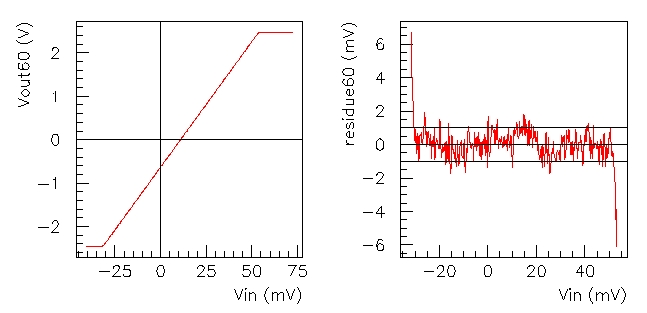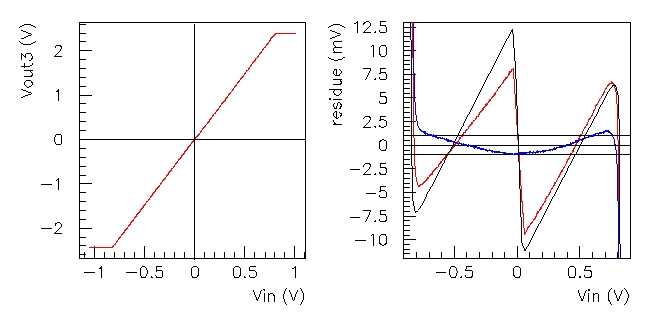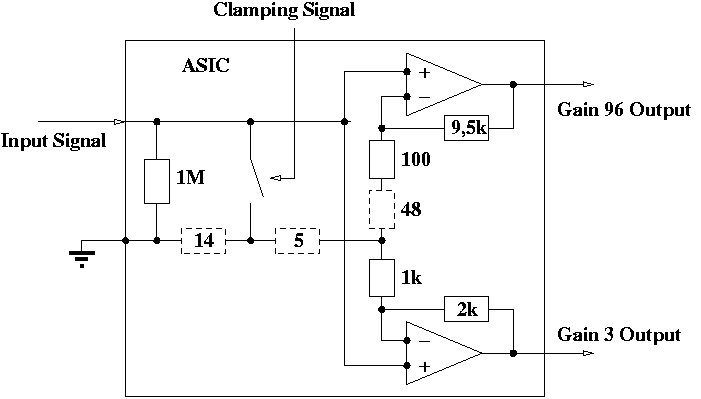# C & S ASIC Linearity Study

The tests of linearity in `DC' mode ( in fact pulses lasting around one millisecond) are part of the performance assessment of the ASIC, and also a way to explore some of the possibilities offered by our pulse generator and our readout system. The first is a 20-bit Digital-Analog Converter with a clock (provided by the Labview acquisition program at this moment), with an output ranging from -2.7 to +2.7 V. We plan to add a faster hardware clocking,in order to simulate CCD pulses of variable heights at an appropriate rate. The second contains two 16-bit Analog to Digital Converters working on a range of  -2.5 to +2.5 V (giving an LSB of 76 µV). With the same clock as 'convert' trigger, their readouts are stored by a 32-bit frame-grabber with a transfer rate of up to 20 MHz.

## Protocol

The protocol for linearity measurement is straightforward thanks to our integrated Labview program : first a DAC ramp is input directly on both ADCs, with the chosen range, step and number of repetitions at each value ; then the ASIC is inserted and the same ramp is applied as ASIC input, while each ADC measures an output. With the averaging, we obtain a standard deviance of 0.21 LSB (16 µV) on the 16-bit ADCs for the low gain and 2 LSB for the high gain (153 µV), which is far more sensitive to input noise. An added twist is that the ASIC was originally designed to function in the -3.5 to 1.5 V range ; the values of the power supply voltages were therefore modified to fit the +/-2.5 V range of the ADCs.

## Results : gain, offset, residues

• The linear fits on the non-saturated parts of the response curves give respectively :
• For the high gain channel : a gain of 57.9 and an offset of -575 mV
• For the low gain channel : a gain of 2.96 and an offset of 5.3 mV ; and after correction a gain of 2.98 and the same offset of 5.3 mVThe plot of the residues from the linear fits is the key to judging the linearity of the amplifiers. In the case of the high gain amplifier, the residues show patterns generated by the ADC ; no deviation from linearity can be attributed to the amplifier. However, in the case of the low gain, the plot shows a clear pattern in the deviation from the linear fitting. A partial fit on each branch of that pattern shows a gain of 2.97 in the negative part, 2.98 in the positive part and only 2.75 in the central part of the +/-2.5 V interval. It should be noted that the different segments match exactly the different modes of the high gain channel, hence the idea to explain this apparent break in linearity by a coupling through the grounding parasitic resistor discussed in the previous section. If there is a resistance between the point where the resistor bridges of both gains are joined and the ground pad (see details on the schematic below), each output should create an additional voltage on the feedback input of the other amplifier.The shape of the low gain residue graph was reproduced by simulating such a configuration (black line in the residues graph). To eliminate this coupling effect from our data and look at how the amplifier would work without those parasitic resistors, we calculated a 'parasitic voltage', created by the current coming from the high gain resistor bridge through a part of the parasitic resistor. Then we calculated the effective input and output voltages by subtracting this parasitic voltage to the measured inputs and outputs. With a common resistor of 19 Ohm, out of a total 67 Ohm on the high gain channel, this operation effectively eliminated the coupling pattern (blue line in the residues graph). This brought the residues down to a reasonable level compared to the 12-bit ADC that will be used for the integration into a readout chain. However, the remaining non-linearity is not yet understood.